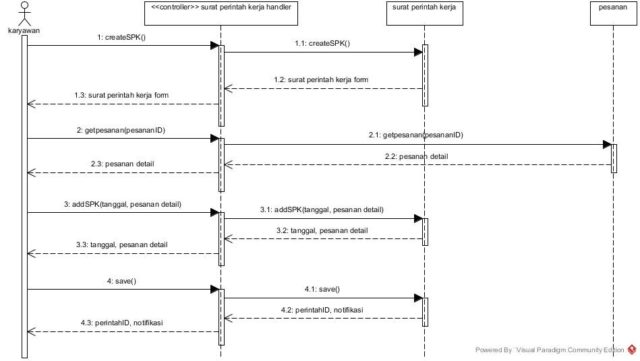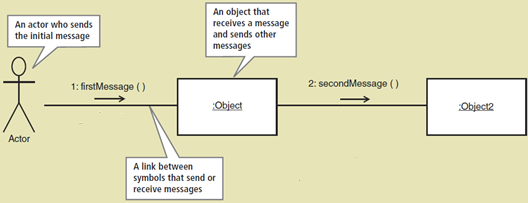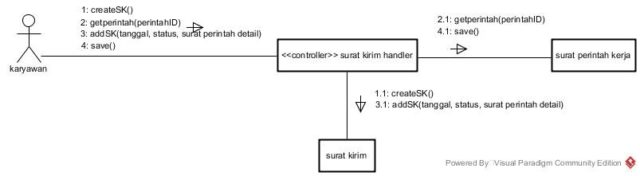# Introduction to Communication Diagram

Communication diagram is one of the UML diagram that can also be called as interaction diagram. This diagram is similar to sequence diagram that is used when we are still designing the system flow in fulfilling the business process requirements. Communication diagram also shows the interactions between classes and actor in the system. The difference with sequence diagram is this diagram shows only the input messages or the messages that are sent by the actor and other objects for a specific use case. Communication diagram has partly same notations with sequence diagram, therefore the actor, objects/classes, and messages can be recalled from a diagram called first-cut sequence diagram. Hereby is the example of first-cut sequence diagram to refresh our memory (first-cut sequence diagram for create work order (surat perintah kerja):As we can see, in the first-cut sequence diagram, there are only actor, handler and objects. We use those notations in the communication diagram. Hereby is the explanation of each notations used in communication diagram:The differences are the connecting line between the actor and objects and no return messages. We draw the connecting line by matching it with the flow of messages in the first-cut sequence diagram. The first object is replaced by the handler based on the specific use case we are working on. The conclusion of making this diagram is by referencing to the first-cut sequence diagram and used for making an overview of the objects and actor that work together. Below is the example of communication diagram by referencing to the first-cut sequence diagram example above (communication diagram for create work order (surat perintah kerja):Amelia Anggraini